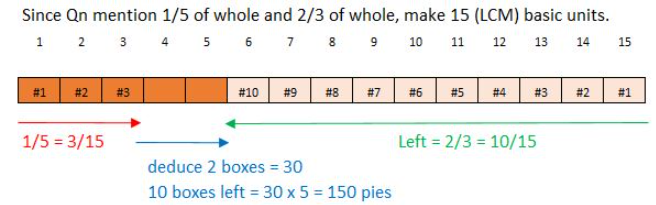# Question

Need help on the above q.

Need help on the above q

Q1.The particpants of the quiz are divided equally into 2 groups.

in the first group, there are 20 more boys than girls.

in the second group, there are 12 more girls than boys.

45% of all participants are girls.

How many participants are boys?

q2. Suyin baked some pies. She gave 1/5 of them to her relatives and 30 of them to her friends. She was left with 2/3 of the pies. She packed these into 18 boxes . Some boxes contained 6 pies while the rest contained 12.

a) how many pies weee packed into 18 boxes?

b) how many boxes contained 6 pies?

Source: PSLE 2012 Maths

Q1) Frankly, all I did was summarising the data before I know what method to use and the results just “pops out” this way. You should deduce the same thing if you draw models of 2 different shapes for group 1 and 2 respectively.

Girls                Boys                      Diff

Group 1         u                    u+20

Group 2        p+12               p

Total          u+p+12         u+p+20                  8 —> 10%(diff of 55% boys and 45% girls)

Boys:   44 <— 55%                Corrected

0 Replies 1 Like ✔Accepted Answer

Q1. The participants of the quiz are divided equally into 2 groups. In the first group, there are 20 more boys than girls. In the second group, there are 12 more girls than boys. 45% of all participants are girls. How many participants are boys?

first group
boys ——- 1u + 20
girls ——- 1u

second group
boys ——- 1p
girls ——- 1p + 12

1u + 1p + 12 ——- 45% (total girls)
1u + 20 + 1p ——- 100% – 45% = 55% (total boys)
55% – 45% = 10% ——- 20 – 12 = 8
55% ——- (8/10) x 55 = 44

Ans : 44 boys.

Q2. Suyin baked some pies. She gave 1/5 of them to her relatives and 30 of them to her friends. She was left with 2/3 of the pies. She packed these into 18 boxes. Some boxes contained 6 pies while the rest contained 12. (a) How many pies were packed into 18 boxes? (b) How many boxes contained 6 pies?

(a)
total pies ——- 15u
(1/5) x 15u = 3u (relatives)
15u – 3u – 30 = 12u – 30 ——- (2/3) x 15u = 10u
12u – 10u = 2u ——- 30
1u ——- 30/2 = 15
10u ——- 150

(b)
18 x 6 = 108 (Assuming all 18 boxes contained 6 pies)
150 – 108 = 42 (pies in boxes of 12)
12 – 6 = 6
42/6 = 7 (boxes contained 12 pies)
18 – 7 = 11
or
18 x 12 = 216 (Assuming all 18 boxes contained 12 pies)
216 – 150 = 66
66/6 = 11

Ans : (a) 1566/6 = 110 pies; (b) 11 boxes.

Thanks

Welcome

0 Replies 0 Likes

Q2)

1 whole  —> take away 1/5 and 30      —-> left 2/3

1 whole  —> take away 3/15 and 30    —-> left 10/15 (LCM of 3 and 5 is 15)

so  30 is 2/15 (balance fraction)

10/15 –> 30 x 5 = 150 pies (Ans a)

Part 2: Assumption method

If all 18 boxes contains 6 pies each –> 18×6=108 pcs

150-108=42 (big diff)

12-6 = 6 (small diff)

42/6 = 7 (must exchange 7 small boxes into big boxes)

18-7 = 11 small boxes of 6 pies (Ans b)

Check: 11 x 6 + 7 x 12 = 150 ok

Thanks Sahm.

for q2)

is this method acceptable?

Give away =1u+30

left= 4u-30

1u+30 = 1p——eq1

4u-30 =2p ——eq2

as they indicated 2/3 are left. As such that I had the equation.

make eq1 =eq2

eq1 x2 throughout

eq1;> 2u+60 =2p

4u-30=2u+60

4u-2u=60+30

2u=90

1u=45

4u-30=4×45-30

=150